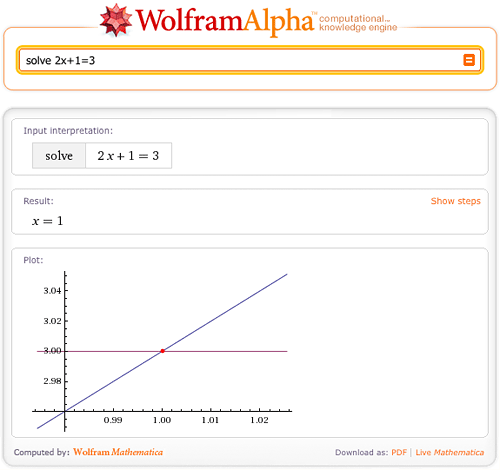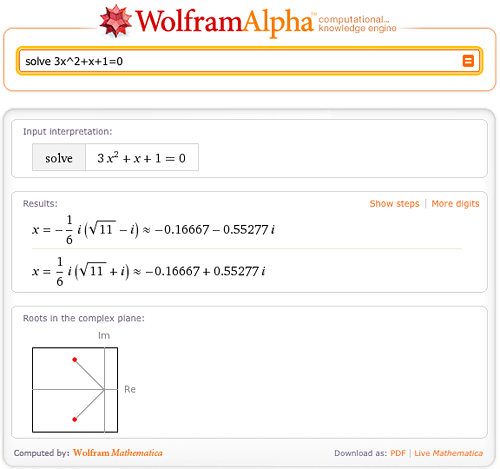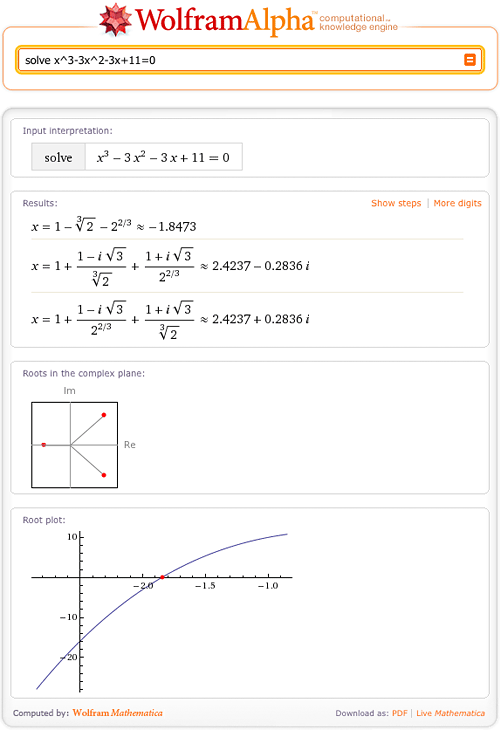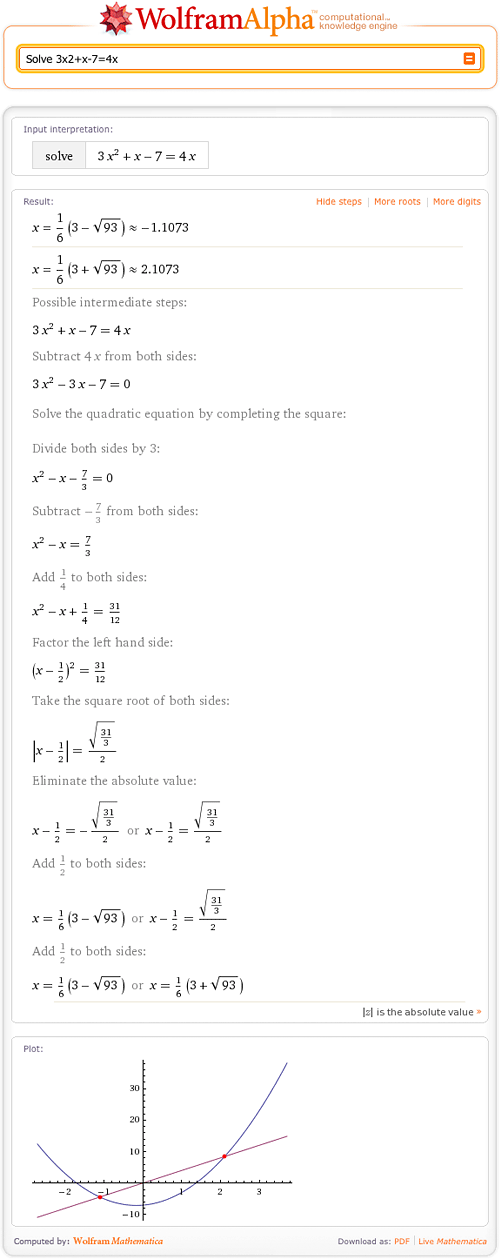TAG: Polynomials
January 14, 2010–

Need a tutor for solving equations? Solving equations is just one of hundreds of mathematical tasks that can be done using Wolfram|Alpha. Wolfram|Alpha can solve equations from middle school level all the way through college level and beyond. So next time you are stumped on an equation, consult Wolfram|Alpha for a little help.

Let’s start with the simpler stuff. Wolfram|Alpha can easily solve linear and quadratic equations, and even allows you to view a step-by-step solution of each problem.What if the roots of the equation are complex? No worries; Wolfram|Alpha has no trouble solving equations over the complex plane.Wolfram|Alpha can also solve cubic and quartic equations in terms of radicals.Of course, some solutions are too large or cannot be represented in terms of radicals; Wolfram|Alpha will then return numerical solutions with a “More digits” button. More »

December 1, 2009–

## July 17, 2019 Update: Step-by-step solutions has been updated! Learn more.

Have you ever given up working on a math problem because you couldn’t figure out the next step? Wolfram|Alpha can guide you step by step through the process of solving many mathematical problems, from solving a simple quadratic equation to taking the integral of a complex function.

When trying to find the roots of 3x2+x–7=4x, Wolfram|Alpha can break down the steps for you if you click the “Show steps” button in the Result pod.As you can see, Wolfram|Alpha can find the roots of quadratic equations. Wolfram|Alpha shows how to solve this equation by completing the square and then solving for x. Of course, there are other ways to solve this problem! More »